ClockWork 17
Set 17 (20 Questions)
(Tell time to the nearest quarter hour and know relationships of time, e.g., minutes in an hour, days in a month, weeks in a year. Determine the duration of intervals of time in hours, e.g., 11 a.m. to 4 p.m. Carry out simple conversions within a system of measurement, e.g., centimeters and meters, hours and minutes.)
From Mr. Anker Tests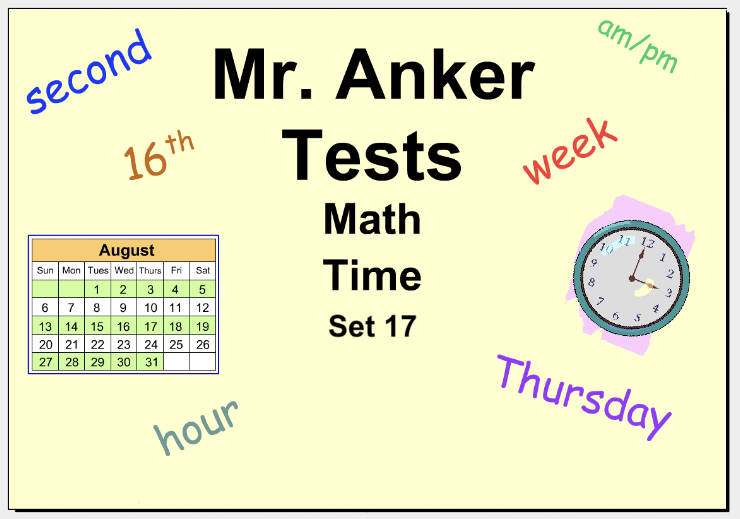1. How many days are there in two weeks?
1 point
2. Which is the longest period of time? *
1 point
3. Which is equal in length to 60 minutes? *
1 point
4. How many months are there in one and a half years? *
1 point
5. Which is the last month in a calendar year? *
1 point
6. Which month of the year has the fewest days? *
1 point
7. The year 2020 was a "leap year" because its calendar had 366 days. Leap years occur every four years. Which of the choices below was not a leap year? *
1 point
8. What day follows Wednesday each week? *
1 point
9. How many times does a clock strike 12:00 each day? *
1 point
10. Which of these times occurs at night? *
1 point
11. Which of these times is one when you would typically be at school on a weekday? *
1 point
12. How many hours pass from 6:00 pm to 1:00 am? *
1 point
13. How much time passes between 9:00 am and 4:30 pm? *
1 point
14. How many hours are there in two full days? *
1 point
15. Which is the shortest amount of time? *
1 point
16. Which of these dates is a Tuesday in August? *
1 point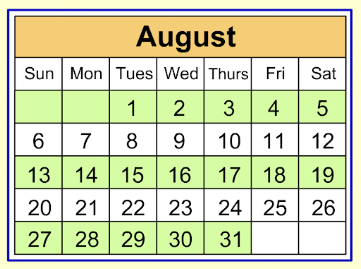17. How many Wednesdays are there in August? *
1 point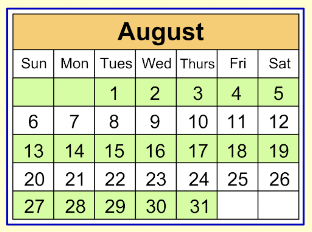18. With which day of the week will the following September begin? *
1 point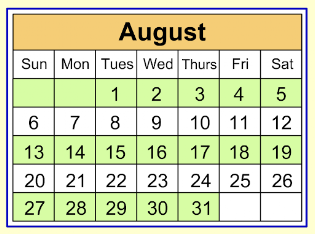19. What is the date of the third Saturday in August? *
1 point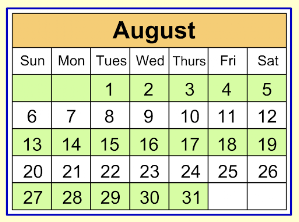20. How much time elapses from 3:45 am to 5:47 am? *
1 point